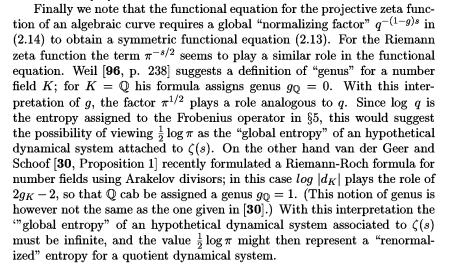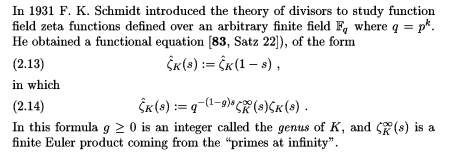number theory and entropy

K.H. Knuth, "Deriving laws from ordering relations", in G.J. Erickson, Y. Zhai (eds.), Bayesian Inference and Maximum Entropy Methods in Science and Engineering, AIP Conference Proceedings 707 (2003) 204–235

[author's description:] "In this paper I show that bi-valuations defined on distributive lattices give rise to a sum rule, a product rule and a Bayes' theorem analog that are most familiar in the realm of Bayesian probability theory. This work is a generalization of Richard T. Cox's derivation of probability theory from Boolean algebra by defining degrees of implication. However, here I show that the potential for application is much greater than previously envisioned. The Möbius function for the distributive lattice gives rise to Gian-Carlo Rota's inclusion-exclusion relation, which is responsible for the form of many laws familiar from areas of study as diverse as probability theory, number theory, geometry, information theory, and quantum mechanics."

K.H. Knuth, "Lattice duality: The origin of probability and entropy", Neurocomputing 67 C (2005) 245-274

[author's description:] "This paper shows how a straight-forward generalization of the zeta function of a distributive lattice gives rise to bi-valuations that represent degrees of belief in Boolean lattices of assertions and degrees of relevance in the distributive lattice of questions. The distributive lattice of questions originates from Richard T. Cox's definition of a question as the set of all possible answers, which I show is equivalent to the ordered set of down-sets of assertions. Thus the Boolean lattice of assertionns is shown to be dual to the distributive lattice of questions in the sense of Birkhoff's Representation Theorem. A straightforward correspondence between bi-valuations generalized from the zeta functions of each lattice give rise to bi-valuations that represent probabilities in the lattice of assertions and bi-valuations that represent entropies and higher-order informations in the lattice of questions."

A.H. Chamseddine, A. Connes and W.D. van Suijlekom, "Entropy and the spectral action" (preprint 09/2018)

[abstract:] "We compute the information theoretic von Neumann entropy of the state associated to the fermionic second quantization of a spectral triple. We show that this entropy is given by the spectral action of the spectral triple for a specific universal function. The main result of our paper is the surprising relation between this function and the Riemann zeta function. It manifests itself in particular by the values of the coefficients $c(d)$ by which it multiplies the $d$ dimensional terms in the heat expansion of the spectral triple. We find that $c(d)$ is the product of the Riemann xi function evaluated at $-d$ by an elementary expression. In particular $c(4)$ is a rational multiple of $\zeta(5)$ and $c(2)$ a rational multiple of $\zeta(3)$. The functional equation gives a duality between the coefficients in positive dimension, which govern the high energy expansion, and the coefficients in negative dimension, exchanging even dimension with odd dimension."

P. Kumar, P.C. Ivanov, H.E. Stanley, "Information entropy and correlations in prime numbers"

[abstract:] "The difference between two consecutive prime numbers is called the distance between the primes. We study the statistical properties of the distances and their increments (the difference between two consecutive distances) for a sequence comprising the first 5 x 107 prime numbers. We find that the histogram of the increments follows an exponential distribution with superposed periodic behavior of period three, similar to previously-reported period six oscillations for the distances."

Nature article on this research (24/03/03)

S.W. Golomb, "Probability, information theory, and prime number theory", Discrete Mathematics 106-107 (1992) 219-229

[abstract:] "For any probability distribution D = {\alpha(n)} on Z+, we define. . . the probability in D that a 'random' integer is a multiple of m; and . . . the probability in D that a 'random' integer is relatively prime to k. We specialize this general situation to three important families of distributions . . . Several basic results and concepts from analytic prime number theory are revisited from the perspective of these families of probability distributions, and the Shannon entropy for each of these families is determined."

C. Bonanno and M.S. Mega, "Toward a dynamical model for prime numbers" Chaos, Solitons and Fractals 20 (2004) 107-118

[abstract:] "We show one possible dynamical approach to the study of the distribution of prime numbers. Our approach is based on two complexity methods, the Computable Information Content and the Entropy Information Gain, looking for analogies between the prime numbers and intermittency."

The main idea here is that the Manneville map Tz exhibits a phase transition at z = 2, at which point the mean Algorithmic Information Content of the associated symbolic dynamics is n/log n. n is a kind of iteration number. For this to work, the domain of Tz [0,1] must be partitioned as [0,0.618...] U [0.618...,1] where 1.618... is the golden mean.

The authors attempt to exploit the resemblance to the approximating function in the Prime Number Theorem, and in some sense model the distribution of primes in dynamical terms, i.e. relate the prime number series (as a binary string) to the orbits of the Manneville map T2. Certain refinements of this are then explored.

"We remark that this approach to study prime numbers is similar to the probabilistic approach introduced by Cramér...that is we assume that the [binary] string [generated by the sequence of primes]...is one of a family of strings on which there is a probability measure..."

E. Canessa, "Theory of analogous force on number sets" (preprint 07/03)

[abstract:] "A general statistical thermodynamic theory that considers given sequences of [natural numbers] to play the role of particles of known type in an isolated elastic system is proposed. By also considering some explicit discrete probability distributions px for natural numbers, we claim that they lead to a better understanding of probabilistic laws associated with number theory. Sequences of numbers are treated as the size measure of finite sets. By considering px to describe complex phenomena, the theory leads to derive a distinct analogous force fx on number sets proportional to $(\fract{\partial p_{x}}{\partial x})_{T}$ at an analogous system temperature T. In particular, this yields to an understanding of the uneven distribution of integers of random sets in terms of analogous scale invariance and a screened inverse square force acting on the significant digits. The theory also allows to establish recursion relations to predict sequences of Fibonacci numbers and to give an answer to the interesting theoretical question of the appearance of the Benford's law in Fibonacci numbers. A possible relevance to prime numbers is also analyzed."

Informational theoretic entropy is defined in this setting in part II.B.

A.I. Aptekarev, J.S. Dehesa, A. Martinez-Finkelshtein, R. Yanez, "Discrete entropies of orthogonal polynomials" (preprint 10/2007)

[abstract:] "Let $p_n$ be the $n$-th orthonormal polynomial on the real line, whose zeros are $\lambda_j^{(n)}$, $j=1, ..., n$. Then for each $j=1, ..., n$, $$\vec \Psi_j^2 = (\Psi_{1j}^2, ..., \Psi_{nj}^2)$$ with $$\Psi_{ij}^2= p_{i-1}^2 (\lambda_j^{(n)}) (\sum_{k=0}^{n-1} p_k^2(\lambda_j^{(n)}))^{-1}, \quad i=1, >..., n,$$ defines a discrete probability distribution. The Shannon entropy of the sequence $\{p_n\}$ is consequently defined as $$\mathcal S_{n,j} = -\sum_{i=1}^n \Psi_{ij}^{2} \log (\Psi_{ij}^{2}) .$$ In the case of Chebyshev polynomials of the first and second kinds an explicit and closed formula for $\mathcal S_{n,j}$ is obtained, revealing interesting connections with the number theory. Besides, several results of numerical computations exemplifying the behavior of $\mathcal S_{n,j}$ for other families are also presented."

P. Tempesta, "Group entropies, correlation laws and zeta functions" (preprint 05/2011)

[abstract:] "The notion of group entropy is proposed. It enables to unify and generalize many different definitions of entropy known in the literature, as those of Boltzmann–Gibbs, Tsallis, Abe and Kaniadakis. Other new entropic functionals are presented, related to nontrivial correlation laws characterizing universality classes of systems out of equilibrium, when the dynamics is weakly chaotic. The associated thermostatistics are discussed. The mathematical structure underlying our construction is that of formal group theory, which provides the general structure of the correlations among particles and dictates the associated entropic functionals. As an example of application, the role of group entropies in information theory is illustrated and generalizations of the Kullback–Leibler divergence are proposed. A new connection between statistical mechanics and zeta functions is established. In particular, Tsallis entropy is related to the classical Riemann zeta function."

T. Benoist, N. Cuneo, V. Jakšić and C.-A. Pillet, "On entropy production of repeated quantum measurements, II: Examples" (preprint 12/2020)

[abstract:] "We illustrate the mathematical theory of entropy production in repeated quantum measurement processes developed in a previous work by studying examples of quantum instruments displaying various interesting phenomena and singularities. We emphasize the role of the thermodynamic formalism, and give many examples of quantum instruments whose resulting probability measures on the space of infinite sequences of outcomes (shift space) do not have the (weak) Gibbs property. We also discuss physically relevant examples where the entropy production rate satisfies a large deviation principle but fails to obey the central limit theorem and the fluctuation-dissipation theorem. Throughout the analysis, we explore the connections with other, a priori unrelated topics like functions of Markov chains, hidden Markov models, matrix products and number theory."

S. Dwivedi, V. Kumar Singh and A. Roy, "Semiclassical limit of topological Rényi entropy in $3d$ Chern–Simons theory" (preprint 07/2020)

[abstract:] "We study the multi-boundary entanglement structure of the state associated with the torus link complement $S^3\setminus T_{p,q}$ in the set-up of three-dimensional $SU(2)$_k Chern–Simons theory. The focal point of this work is the asymptotic behavior of the Rényi entropies, including the entanglement entropy, in the semiclassical limit of $k\to\infty$. We present a detailed analysis for several torus links and observe that the entropies converge to a finite value in the semiclassical limit. We further propose that the large $k$ limiting value of the Rényi entropy of torus links of type $T_{p,pn}$ is the sum of two parts: (i) the universal part which is independent of $n$, and (ii) the non-universal or the linking part which explicitly depends on the linking number $n$. Using the analytic techniques, we show that the universal part comprises of Riemann zeta functions and can be written in terms of the partition functions of two-dimensional topological Yang–Mills theory. More precisely, it is equal to the Rényi entropy of certain states prepared in topological $2d$ Yang–Mills theory with $SU(2)$ gauge group. Further, the universal parts appearing in the large $k$ limits of the entanglement entropy and the minimum Rényi entropy for torus links $T_{p,pn}$ can be interpreted in terms of the volume of the moduli space of flat connections on certain Riemann surfaces. We also analyze the Rényi entropies of $T_{p,pn}$ link in the double scaling limit of $k\to\infty$ and $n\to\infty$ and propose that the entropies converge in the double limit as well."

This is the concluding paragraph from J. Lagarias, "Number theory zeta functions and dynamical zeta functions", in Spectral Problems in Geometry and Arithmetic (T. Branson, ed.), Contemporary Math. 237 (AMS, 1999) 45-86:(2.13) and (2.14) appear on p.7 in the following paragraph:G. van der Geer and R. Schoof, "Effectivity of Arakelov divisors and the theta divisor of a number field", Selecta Math. (N.S.) 6 (2000) 377-398
 F.K. Schmidt, "Analytische Zahlentheorie in Körpern der Characteristik p", Math. Z. 33 (1931) 1-32
 A. Weil, "Sur l'analogie entre les corps de nombres algébraiques et les corps de functions algébreques", Revue Scient. 77 (1939) 104-106 (also in Collected Papers, Vol. I, Springer-Verlag, pp.236-240)

Prime Numbers and Entropy (Flash applet)

Irish teenager David Doherty has won the 2002 ESAT Young Scientist of the Year Award by proving a conjecture related to the distribution of primes. His continuing research project entitled "The Distribution of the Primes and the Underlying Order to Chaos" seeks to investigate parallels with thermodynamics, entropy, etc.

statistical mechanics and number theory
archive      tutorial      mystery      new      search      home      contact